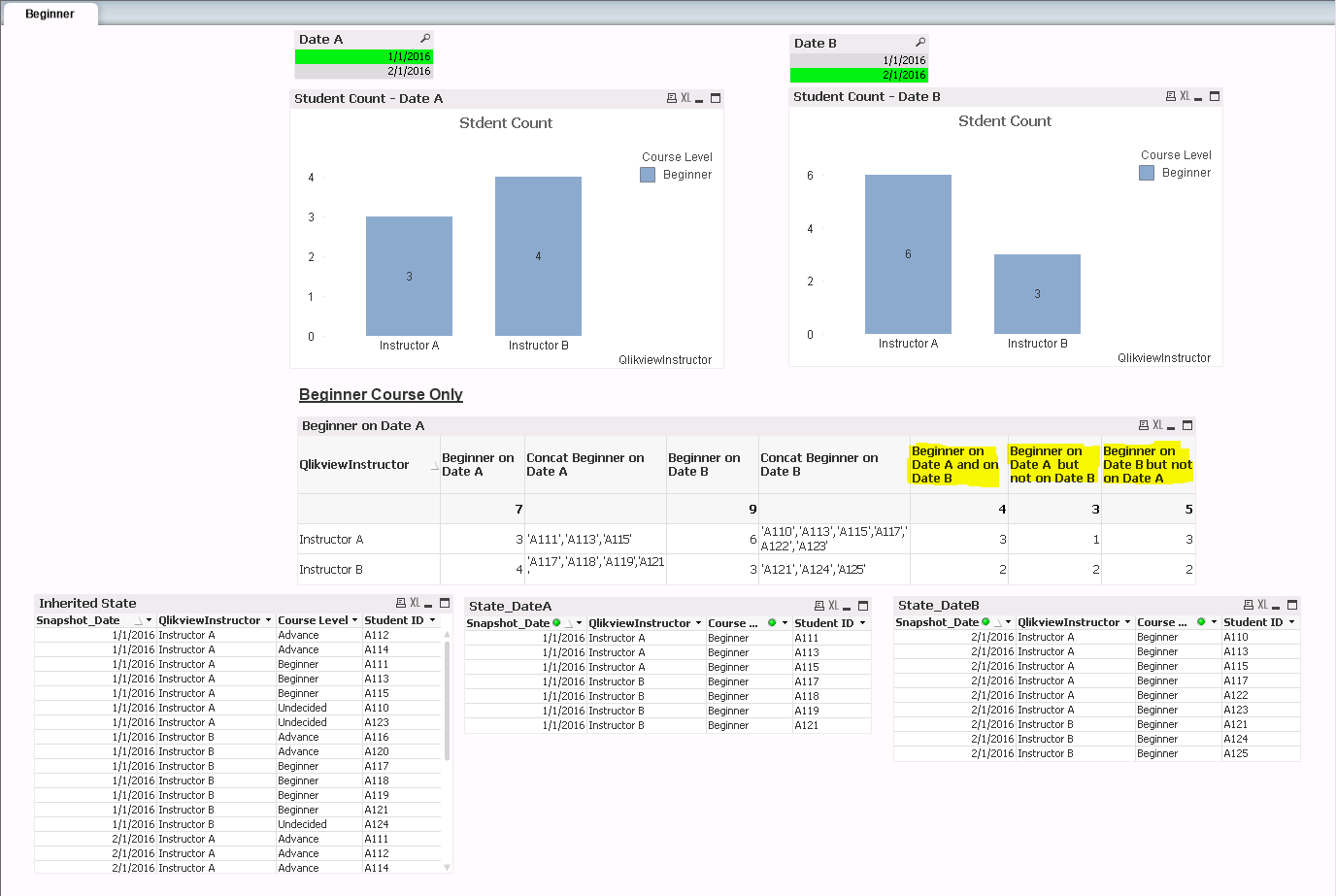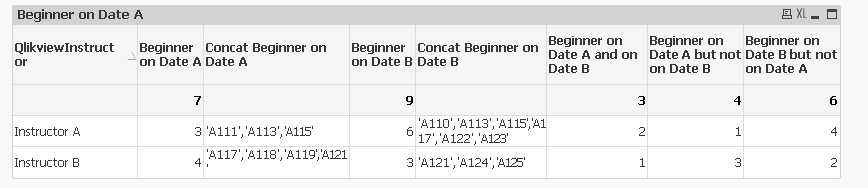# QlikView App Development

Discussion Board for collaboration related to QlikView App Development.

Announcements
Save the Date: QlikWorld Online, June 24-25, 2020. Free global virtual event for data integration and data analytic gurus. Register TodayNot applicable

## Include Dimension Value in Set Analysis Expression

I refer to the comment “a set is only generated once for the entire table, not once per value of the dimensions” in the January 4, 2011 Evaluating "sets" in the context of a dimension post by Gordon Savage. I am hoping that someone has devised a simplified work around for this problem or at least someone can assist me with the problem described below. Attached are two files:

1. A Qlikview dashboard with the charts/tables I would like to create
2. An xlsx file with the sample data

The Qlikview dashboard is supposed to function as follows:

• There are two alternate state: One for  Date A and the other for Date B. The user should use the list boxes to select the values for Date A and for Date B
• The straight table below should shows for the Beginner course only:
1. Number of students registered with each Instructor on Date A
2. Number of students registered with each Instructor on Date B
3. For each Instructor, the number of students registered on both Date A and Date B
4. For each Instructor, the number of students registered on Date A, but not Date B
5. For each Instructor, the number of students registered on Date B, but not Date B

In the example shown in the screenshot, the values in the last three columns are incorrect. Refer to columns 3 and 5 with the concatenated Student IDs.

3. The number of students registered on both Date A and Date B for Instructor A and B should be 2 and 1 respectively

4. The number of students registered on Date A but not on Date B for Instructor A and B should be 1 and 3 respectively

5. The number of students registered on Date B but not on Date A for Instructor A and B should be 4 and 2 respectively

I will be extremely grateful if someone can help me solve this problem.

Thanks

MariaTags (4)
1 Solution

Accepted Solutions
HighlightedMVP

## Re: Include Dimension Value in Set Analysis Expression

Try like this

[Student ID],

QlikviewInstructor,

[Course Level],

AutoNumber(QlikviewInstructor&[Student ID]) as Key

FROM

[Qlikview Course Enrolment.xls]

(biff, embedded labels, table is Sheet1\$);Beginner on Date A and on Date B

= Count(DISTINCT {<Key = P({\$*State_DateA<[Course Level] = {'Beginner'}>})*P({\$*State_DateB<[Course Level] = {'Beginner'}>})>} [Student ID])

Beginner on Date A but not on Date B

=COUNT(DISTINCT {<Key = P({\$*State_DateA<[Course Level] = {'Beginner'}>}) - P({\$*State_DateB<[Course Level] = {'Beginner'}>})>} [Student ID])

Beginner on Date B but not on Date A

=COUNT(DISTINCT {<Key = P({\$*State_DateB<[Course Level] = {'Beginner'}>}) - P({\$*State_DateA<[Course Level] = {'Beginner'}>})>} [Student ID])

3 Replies
HighlightedMVP

## Re: Include Dimension Value in Set Analysis Expression

Try like this

[Student ID],

QlikviewInstructor,

[Course Level],

AutoNumber(QlikviewInstructor&[Student ID]) as Key

FROM

[Qlikview Course Enrolment.xls]

(biff, embedded labels, table is Sheet1\$);Beginner on Date A and on Date B

= Count(DISTINCT {<Key = P({\$*State_DateA<[Course Level] = {'Beginner'}>})*P({\$*State_DateB<[Course Level] = {'Beginner'}>})>} [Student ID])

Beginner on Date A but not on Date B

=COUNT(DISTINCT {<Key = P({\$*State_DateA<[Course Level] = {'Beginner'}>}) - P({\$*State_DateB<[Course Level] = {'Beginner'}>})>} [Student ID])

Beginner on Date B but not on Date A

=COUNT(DISTINCT {<Key = P({\$*State_DateB<[Course Level] = {'Beginner'}>}) - P({\$*State_DateA<[Course Level] = {'Beginner'}>})>} [Student ID])

HighlightedNot applicable

## Re: Include Dimension Value in Set Analysis Expression

Sunny,

Thank you very much. I will try your proposed solution and will get back to you.

Also, I gave a very simplified version of my data set and the straight table that I am required to develop. My actual data set requires that I have a drill-down group as the dimension.  That is, suppose instead of a simple dimension as QlikviewInstructor, there was a drill-down comprising fields such as School -> Dept ->  QlikviewInstructor. Will your proposed solution work?

Thanks

Maria

HighlightedMVP

## Re: Include Dimension Value in Set Analysis Expression

The other option would be to use Aggr() function which would work perfectly with a cycle or drill-down dimension, but exact expression will have to be tested out. Can you provide anther sample where you have School and Dept in your application?

Also, if QlikViewInstructor belong to one and only one dept and each department belong to one and only one school, then the above approach might still work for you.